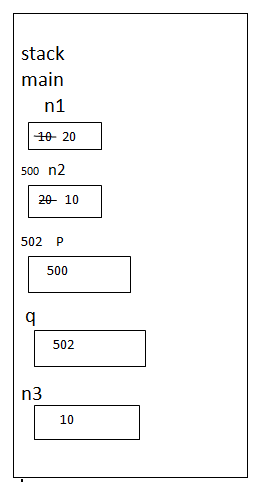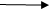Pointers in C++ Language
Ratings:
(4)
Views:349## Pointers

Pointer is a derived data type. A variable of type of pointer is called pointer variable. A pointer variable hold address of memory location

### Advantages of Pointers in C++

Dynamic memory allocation

Allows to share data between functions

Increment the effiecient of the program

Not secured

### Pointer Declaration

Syntax of Pointers in C++ <data type> * variable – name ;              int * * - - >  indirection operator Dereferencing operator Value of given address What is address ?

• If is an unsigned interger value given to each byte of memory.
• Address is a pointer value.

int *a

• The size of pointer variable depends on compiler

16 bit compiler   - - >   2 bytes 32 bit compiler   - - >     4 bytes Example C++ program using Pointers # include <iostream.h> void main() { int *p; char *q; float *r; double *d;   cout << sizeof(p) << endl; cout<<sizeof(q)<<endl; cout<<sizeof(r)<<endl; cout<<sizeof(d)<<endl;   o/p : 4 4                   BCZ turbo c++ 32 bit compiler 4 4

### Write a program to swap two numbers

# include <iostream.h> void main() { int n1,n2; cout<< “\n input any two numbers”; cin>> n1 >> n2; int *p,*q; p=&n1; Q=&n2; int n3; n3=*p;   //  *p - - >   value of 500 *p=*q; // *q - - >   value of 502 *q=n3; cout<<”\n n1=”<<*p; cout<<”\n n2=”<<*q; } o/p : input - - >   10 20After swapping value -> 20 10   Example # include <iostream.h> void main() { int n1,n2; cout<<”\n input any two numbers”; cin>>n1>>n2; int *p,*q; p=&n1; q=&n1; *p=*p+*q;                     // value of 500=value of 500 + value of 500 *q=*p-*q;                                                        =  10+20=  30 *p=*p-*q; cout<<”\n after swapint \n”; cout<<”\n n1=”<<n1; cout<<”\n n2=” <<n2; }     o/p : input two values => 10 20 After swapping => 20 10

### Dynamic memory Allocation

Allocating memory during the execution if runtime program is called as dynamic memory allocation

It avoiding wastage of memory

Increase efficiency of program

### What is static memory allocation

Size of memory allocated by program, known at compile time on before executing program. is called static memory allocation.

### What is dynamic memory allocation

Size of memory allocated by program is not known at compile time but known at runtime is called dynamic memory allocation

new

delete c++ achieves dynamic memory allocation using 2 keywords

### new(in c, it is called malloc)

It allocates memory of n bytes in heap area and return address.

Heap area is dynamic memory area, which is manage by c++ program.

syntax : <pointer  - variable – name >=new datatype[size];

size can be constant or variable

size is optional   Texa code area Data areaglobal variable Heap areaDynamic stack arealocal variable are use.

### // wap to add two numbers (allocating memory dynamically)

# include <iostream.h> void main() { int *a,*b,*c; a=new int; b=new int; c=new int; cout<< “\n input any two numbers”; cin>> *a>> *b; *c=*a-*b; cout<<”\n sum is”<<*c; } o/p : input any two numbers.

### Delete:

Delete unreserved memory which is reserved using new operator. delete<pointer-variabel>;

### Difference  betweenMalloc and new

 Malloc new It is a function It is a (operator) keyword It is reserved memory in one block. It is allocates one or more than one block of memory. Malloc does required type casting It doesn’t required type  casting. It does not understand constructor It understand constructor.

### Difference between Delete and Free:

 Delete Free It is a key word It is a function understand destruction It doesn’t understand destructor.

### WAP to for swapping two numbers

# include <iostream.h> void main() { int *a, *b; a=new int; b=new int; cout<<” \n input any two numbers\ n”; cin>> *a>>*b; *a =*a+*b; *b=*a-*b; *a=*a-*b; cout<< “\n After swapping two numbers\n”; cout<< *a << “\t”<<*b; delete a; delete b; cout<< *a; cout<< *b;     Pointer variable which holdsgf an address of memory location whose lifetime is over is called as Dangling pointer. Drawback:

• It splits some logical error.

### NULL

• NULL is a pointer value.
• If pointer variable assign NULL, it does not point to any memory location.